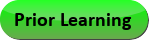Oct 20, 2021
2021-2022 College Catalog
 Select a Catalog 2021-2022 College Catalog 2020-2021 College Catalog [ARCHIVED CATALOG] 2019-2020 College Catalog [ARCHIVED CATALOG]
 HELP 2021-2022 College Catalog Print-Friendly Page (opens a new window)

# MAT 220 - Calculus I [SUN# MAT 2220]

5 Credits, 5 Contact Hours
5 lecture periods 0 lab periods

Introduction to analytical geometry and calculus. Includes limits and continuity, derivatives, applications of the derivative, and integration.

Prerequisite(s): Within the last three years: MAT 187  or MAT 188 , and MAT 189  with a C or better; or required score on the Mathematics assessment exam.
Gen-Ed: Meets AGEC - MATH; Meets CTE - M&S.Course Learning Outcomes
1. Evaluate limits of functions.
2. Differentiate functions and apply derivatives.
3. Determine antiderivatives of functions and apply the Fundamental Theorem of Calculus.

Performance Objectives:
1. Evaluate certain limits analytically, and estimate other limits numerically and/or graphically.  These limits include double-sided, one-sided, and limits at infinity.
2. Use the definition of continuity to identify points and types of discontinuity of functions defined analytically or graphically.
3. Use the definition of the derivative to calculate the exact derivative of certain functions and/or estimate the value of the derivative at a point.
4. Sketch the derivative of a function defined graphically.
5. Explain the meaning of the derivative in an applied situation using appropriate units.
6. Calculate derivatives, explicitly and implicitly, of algebraic combinations of polynomial, radical, exponential, logarithmic, trigonometric, and inverse trigonometric function.
7. Determine the linear approximation of a function defined analytically, numerically, or graphically.
8. Solve related rates problems.
9. Calculate higher order derivatives of algebraic combinations of polynomial, radical, exponential, logarithmic, trigonometric, and inverse trigonometric functions.
10. Estimate small changes in a function using differentials.
11. Use the 1st derivative to identify critical points and intervals of increase and decrease.
12. Identify the type and location of extrema using 1st and/or 2nd derivative tests.
13. Use the 2nd derivative to identify intervals of upward and downward concavity and inflection points.
14. Sketch graphs of algebraic and transcendental functions using information obtained from derivatives and other analyses.
15. Evaluate a variety of indeterminate forms using L’Hopital’s Rule.
16. Solve a variety of optimization problems using derivatives.
17. Find antiderivatives of polynomial, exponential, and some rational and trigonometric functions.
18. Solve applied problems requiring the use of antiderivatives such as acceleration, velocity, and position problems.
19. Sketch the graph of a possible antiderivative of a function defined graphically.
20. Use finite sums to estimate the definite integral of functions defined numerically, graphically or analytically.  Estimate techniques should include some of the following: left/right hand, trapezoid, and midpoint rules.
21. Interpret the definite integral in an applied situation using appropriate units.
22. Evaluate definite integrals using the Fundamental Theorem of Calculus.
23. Calculate the area beneath the graph of a function using the definite integral.
24. Use the Fundamental Theorem of Calculus to demonstrate that differentiation and integration are inverse operations.
25. Use the technique of “substitution” to evaluate definite and indefinite integrals.

Optional Objectives:

26. Calculate derivatives of hyperbolic functions.

27. Calculate derivatives using logarithmic differentiation.

28. Use calculus to investigate the graphs of and distinguishing characteristics of families of functions.

29. Identify the condition where the Mean Value Theorem and/or the Extreme Value Theorem apply.

30. Estimate the solution of an equation using Newton’s Method.

31. Calculate areas between curves and simple applications problems using definite integrals.

Outline:
1. Limits and Continuity
1. 2-sided
2. 1-sided
3. Limits involving infinity
4. Definition of continuity
5. Points and types of discontinuity
2. Derivatives
1. Definition of the derivative
1. Estimate the value of the derivative
2. Calculate exact derivatives
2. Meaning of the derivative
3. Differentiation Rules
1. Power rule
2. Product rule
3. Quotient rule
4. Chain rule
4. Derivatives of transcendental functions
1. Trigonometric functions
2. Inverse trigonometric functions
3. Exponential functions
4. Logarithmic functions
5. Hyperbolic functions (optional)
6. Logarithmic differentiation (optional)
5. Implicit differentiation
6. Higher order derivatives
3. Applications of the Derivative
1. Related rates
2. Linear approximations
3. Differentials
4. Curve sketching
1. Intervals of increase and decrease
2. Extrema
3. Intervals of concavity
4. Points of inflection
5. Families of functions (optional)
6. Optimization
7. Antiderivatives
1. Polynomial functions
2. Exponential functions
3. Rational functions
4. Trigonometric functions
5. Applied problems
8. L’Hopital’s Rule
9. Mean Value Theorem (optional)
10. Newton’s Method (optional)
4. Integration
1. Definition of the definite integral
2. Estimate the definite integral
3. Fundamental Theorem of Calculus
4. Indefinite integrals
5. Area under curves
6. Integration by substitution
7. Area between curves (optional)

Effective Term:
Spring 2017